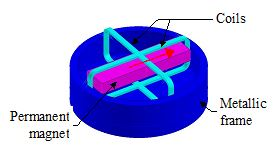# 3D example: Rotating motion (technical paper)

## Foreword

This paragraph is a summary of cases, treated in detail in the technical document: " 3D Rotating motion Tutorial ".

The files relating to the studied cases, are accessible via the supervisor, Open Example context.

## Study carried out

The study proposed in the 3D tutorial " Rotating motion " is the study of a transducer used to display automobile speed.

## Studied device

The studied device, represented on the figure below, includes the following elements:

• a metallic frame (non conductive)
• a permanent magnet magnetized along its length
• two fixed rectangular flat coils, perpendicular to each other, placed around the magnet## Operation

The operation of the device is explained below:

• A speed sensor measures the automobile speed and sends the information expressed by electric currents to the transducer coils.
• The position of the moving permanent magnet is a function of the value of the current in the two coils, and thus, the automobile's speed is displayed by a needle fixed with respect to the magnet
• The operation of the device can be considered equivalent to that of a compass.

## Treated cases

The simulations (described in the 3D Technical Paper) are carried out in Flux by means of the study of four cases:

• case 1: parametric study without motion (fixed angular position)
• case 2: study with the multi-static model (different angular positions)
• case 3: study with the imposed speed model
• case 4: study with the couple load model

## Case 1

The first case is a parametric study (Magneto Static application):

In this first case, the moving part of the device (the permanent magnet) is not moving.

One is interested in the influence of various parameters: the frame diameter, the relative magnetic permeability of the frame material and the current in the coils. The two coils are supplied with the same current value.

The purpose of this case is to compute the electromagnetic torque and magnetic flux density for different values of the parameters defined above.

## Case 2

The second case is a study with the multi-static model (Magneto Static application):

In this second case ( multi-static model ), the moving part of the device can take various positions (fixed arbitrarily).

One is interested in the computation of the magnetic field for arbitrarily chosen positions . The two coils are supplied with the same current value.

With this model it is possible to evaluate the torque acting on the magnet at different positions. This study determines the equilibrium position of the magnet with respect to the two coils, which is around 45°.

## Case 3

The third case is a study with the imposed speed model (Transient Magnetic application):

In this third case ( imposed speed model ), the moving part of the device (the magnet) is driven by an external device at a constant speed, regardless of the current values through the coils. The coils are not supplied.

The advantage of this model of the field-circuit coupling type is to evaluate the induced voltages in the two coils due to the magnet's motion.

## Case 4

The fourth case is a study with the coupled load model (Transient Magnetic application):

In this fourth case ( couple load model ), the moving part drives an external device, characterized by given values of its mechanical characteristics. The two coils are supplied with the same current value.

The advantage of this model is to study the time variation of the position and speed of the magnet and the time variation of the electromagnetic torque acting on the magnet.